# 2nd PUC Maths Question Bank Chapter 6 Application of Derivatives Ex 6.5

Students can Download Maths Chapter 6 Application of Derivatives Ex 6.5 Questions and Answers, Notes Pdf, 2nd PUC Maths Question Bank with Answers helps you to revise the complete Karnataka State Board Syllabus and score more marks in your examinations.

## Karnataka 2nd PUC Maths Question Bank Chapter 6 Application of Derivatives Ex 6.5

### 2nd PUC Maths Application of Derivatives NCERT Text Book Questions and Answers Ex 6.5

Question 1.
Find the maximum and minimum values, if any, of the following functions given by

(i) f(x) = (2x – 1)2 + 3
f (x) = (2x – 1)2 + 3
For all values of x, f (x) > 3 (2x – 1)2 + 3 > 3
∴ minimum value is 3 when 2x -1 = 0 is x = $$\frac{1}{2}$$ however the function has no maximum values as f (x) → ∞ as |x| ∞

(ii) f (x) = 9x2 + 12x + 2
f (x) = 9x2 + 12x + 2(iii) f (x) = – (x – 1)2 + 10
f (x) = – (x – 1)2 + 10
f(x)= 10 – (x – 1)2
10 – (x – 1)2 < 10
f(x) has a maximum value when x – 1 = 0,x= 1.
how ever f (x) has no minimum value.

(iv) g(x) = x3 + 1
g(x) = x3 + 1 as x → ∞ g (x) → ∞ and a
s -+ – ∞, g (x) ⇒ – ∞
∴ g (x) has neither minimum value nor maximum value.

Question 2.
Find the maximum and minimum values, if any, of the following functions given by

(i) f (x) = |x + 2| – 1
f (x) = |x + 2| – 1
f (x) = |x + 2| – 1 ≥ -1
minimum value is – 1 when x + 2 = 0, x = -2
however it has no maximum value.

(ii) g(x) = – | x + 1| + 3
g(x) = – | x + 1| + 3 = 3,-1 x + 1|
g (x) < 3 v x + 1 = 0
∴ max. value is 3
when x = – 1
how ever no minimum value.

(iii) h(x) = sin (2x) + 5
h(x) = sin (2x) + 5
maximum value of sin 2x = 1 and minimum value is -1
f(x) = 1 + 5 is 1 + 5
∴ max. h (x) = 6 and
min. h (x) = 4.

(iv) f (x) = |sin 4x + 3|
f (x) = |sin 4x + 3|
-1 < sin 4x < 1 ⇒ 3 -1 < sin 4x + 3 < + 1 + 3 + 2′< sin 4 x + 3 < 4
2 < | sin 4x + 3 | < 4
f (x) > 2 and f (x) < 4
min. f (x) = 2 when sin 4x + 3 = 0
max f (x) = 4 when sin 4x + 3 = 0
∴ minimum value is 2 at sin 4x = -1
maximum value is 4 at sin 4x = 1(v) h(x) = x + 1, x ∈ (- 1, 1)
Ans:
h(x) = x + 1, x ∈ (-1,1)
given that x ∈ (-1, 1)
ie. -1 < x < 1
-1 + 1< x + 1 < 1 + 10 < x + 1< 2
∴ x + 1 > 0 or x + 1 < 2
x + 1 > 0 so no minimum value
x + 1 < 2, so no maximum value.

Question 3.
Find the local maxima and local minima, if any, of the following functions. Find also the local maximum and the local minimum values, as the case may be:
(i) f (x) = x2
f (x) = x2
f’ (x) = 2x, f’ (x) = 0
⇒ 2x = 0, x = 0
f'(x)= 2
f'(x)> 0, hence f (x) has minimum value at x = 0
and the minimum value is (0)2= 0.

(ii) g(x) = x3 – 3x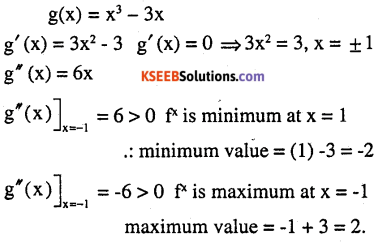(iii) h(x) = sin x + cos x, o < x< $$\frac{\pi}{2}$$(iv) f (x) = sin x – cos x, 0 < x < 2π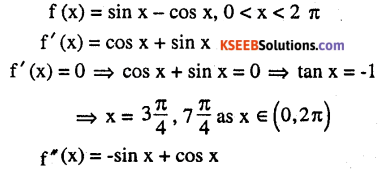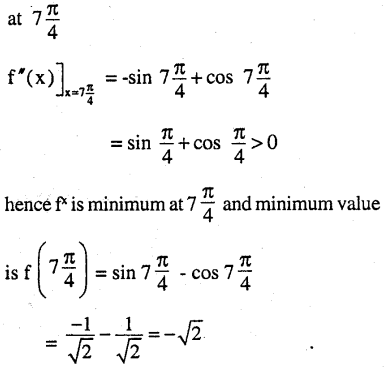(v) f (x) = x3 – 6x2 + 9x + 15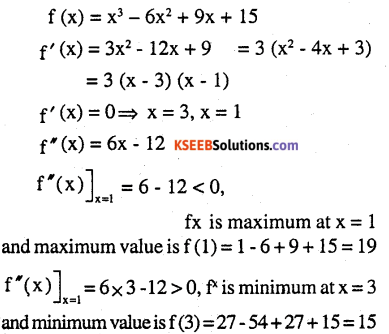(vi) $$g(x)=\frac{x}{2}+\frac{2}{x}, x>0$$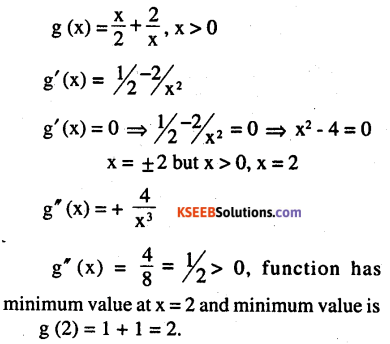(vii) $$g(x)=\frac{1}{x^{2}+2}$$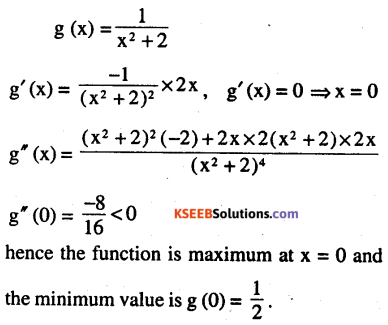(viii) $$f(x)=x \sqrt{1-x}, x>0$$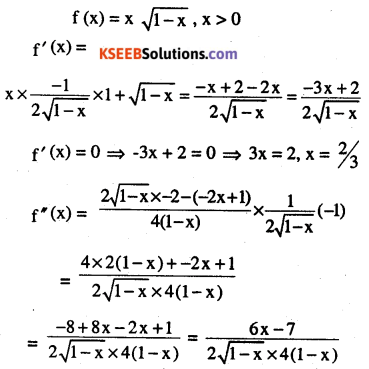Question 4.
Prove that the following functions do not have maxima or minima :

(i) f (x) = ex
f (x) = ex
f’ (x) = ex
f'(x) > 0 ∀ x ∈ R
hence function has no critical point There is no point at which the function is maximum or minimum.(ii) g(x) = log x, x > 0
g (x) = log x, x > 0
g'(x) = $$\frac{1}{x}$$, where x > 0
hence the function has no critical point
∴ There is no point at which the function is maximum or minimum.

(iii) h (x) = x3 + x1 + x +1
h (x) = x3+ x2+ x +1
h’ (x) = 3x2 + 2x + 1
h’ (x) = 0 ⇒ 3x2 + 2x + 1 = 0,
x has no real value, hence there is no critical point.
∴ For no point the function has max. or min. value.

Question 5.
Find the absolute maximum value and the absolute minimum value of the following functions in the given intervals:

(i) f(x) = x3,x ∈ [-2,2]
f (x) = x3,x ∈ [- 2,2]
f’ (x) = 0 ⇒ 3x2 = 0 ⇒ x = 0 for finding the absolute maximum and absolute minimum, we have to evaluate f (0), f (2), f (-2)
F (0) = (0)3 = 0, F (2) = (2)3 = 8,
F (-2) = (-2)3 = – 8
Absolute maximum = 8 and
Absolute minimum = -8
∴ maximum at 2 is 8 and minimum at -2 is – 8.(ii) f (x) = sin x + cos x , x ∈ [0, π](iii) $$f(x)=4 x-\frac{1}{2} x^{2}, x \in[-2,9 / 2]$$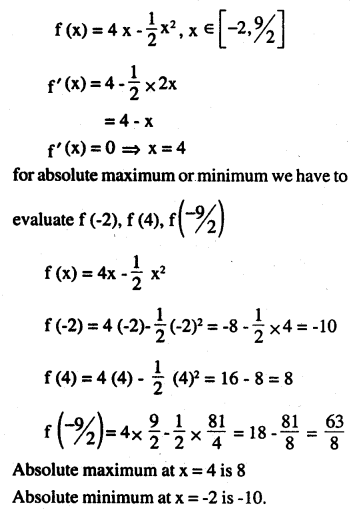(iv) f (x) = (x – 1)2 + 3, x ∈ [-3,1]
f (x) = (x – 1)2 + 3, x ∈ [-3,1]
f'(x) = 2 (x – 1)
f’ (x) = 0 ⇒ (x – 1) = 0
⇒ x = 1 we will evaluate f (-3) and f (1)
f(-3) = (-3 – 1)2 + 3= 16 + 3 = 19
f (1) = (0)2 + 3 = 3
Absolute maximum at x = -3 is 19 and
Absolute minimum x = 1 is 3.

Question 6.
Find the maximum profit that a company can make, if the profit function is given by
p(x) = 41+24x – 18x2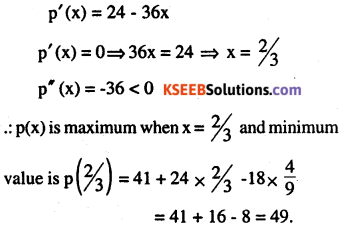Question 7.
Find both the maximum value and the minimum value of
3x4 – 8x3 + 12x2 – 48x + 25 on the interval [0, 3].
f (x) = 3x4 – 8x3 + 12x2 – 48 x + 25
f’ (x) = 12x3 – 24x2 + 24x – 48
f‘(x) = 0 ⇒ 12 (x3 – 2x2 + 2x -4) = 0
⇒ 12 (x2 (x – 2) + 2 (x – 2))
⇒ 12 ( (x – 2) (x2 + 2) ) = 0 (x – 2)(x2 + 2) = 0
⇒ x = 2 but x2 + 2 ≠ 0
The points are f (0), f (2), f (3)
f (x) = 3x4 – 8x3 + 12x2 – 48x + 25
f (0) = 25
f (2) = 3 (16) – 8 (8) + 12 (4) – 48 (2) + 25 = 48 – 64 + 48 – 96 + 25 = -39
f(3) = 3 (34) – 8 (33) + 12 (32) – 48 (3) + 25 = 243 – 216 + 108 – 144 + 25
376 – 360 = 16
∴ maximum of f (x) at x = 0 is 25
minimum of f (x) at x = 2 is – 39.Question 8.
At what points in the interval [0,2π] does the function sin 2x attain its maximum value?Question 9.
What is the maximum value of the function sin x + cos x on $$\left[0, \frac{2}{\pi}\right]$$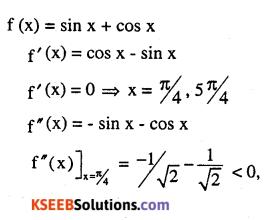Question 10.
Find the maximum value of 2x3 – 24x + 107 in the interval [1, 3]. Find the maximum value of the same function in [-3, -1]
f (x) = 2x3 – 24x +107
f’ (x) = 6x2 – 24 ⇒ x2 = 4, x = ± 2 + 2 s [1,3]
∴ we have to evaluate
f (1). f (2), f (3)
f (1) = 2 (1)3 – 24 x 1 + 107 = 85
f (2) = 2 (2)3– 24 x 2+ 107 = 75
f(3) = 2(3)3-24 x 3 + 107 = 89
maximum at x = 3 and miximum value is 89 minimum at x = 2, is 75
Now the interval is [-3, -1]
∴ points are -3, -2, -1
f (-3) = 2 (-3)3 – 24 (-3) + 107 = 125
f (-2) = 2 (-2)3 – 24 (-2) + 107
= 2 (-8+ 48 + 107 = 139 = 139
f (-1) = 2 (-1) 3-24 (-1) + 107 = 129
maximum at -2 is 139
minimum at (-3) is 125.Question 11.
It is given that at x = 1, the function x4 – 62x2 + ax + 9 attains its maximum value, on the interval [0,2]. Find the value of a.
f (x) = x4 – 62x2 + ax + 9
f'(x) = 4x3 – 124x + a
at x = 1, the function has maximum value
∴ f'(1) = 0 ⇒ 4 (1)2 -124 (1) + a = 0, a = 120
∴ when a = 120, the function attains maximum value.

Question 12.
Find the maximum and minimum values of x + sin 2x on [0,2π]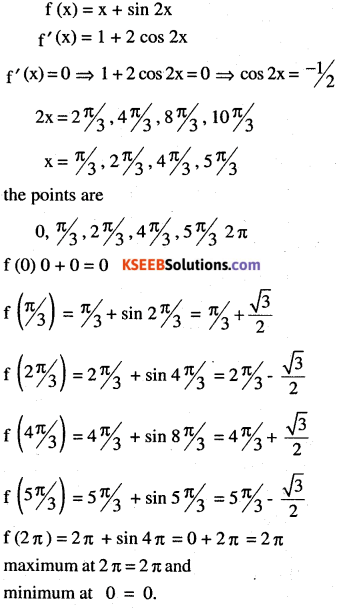Question 13.
Find two numbers whose sum is 24 and whose product is as large as possible.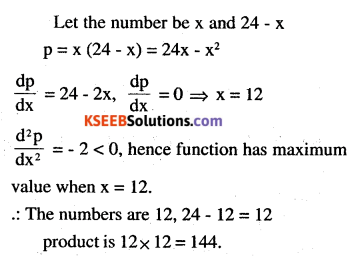Question 14.
Find two positive numbers x and y such that x + y = 60 and xy3 is maximum.
x + y = 60 ⇒ y = 60 – x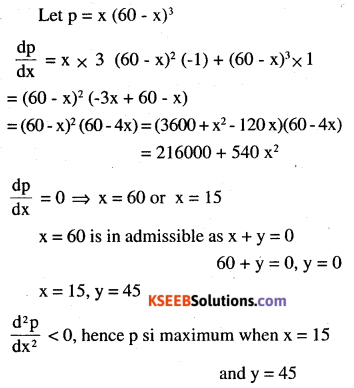Question 15.
Find two positive numbers x and y such that their sum is 35 and the product x2 y5 is a maximum.Question 16.
Find two positive numbers whose sum is 16 and the sum of whose cubes is minimum.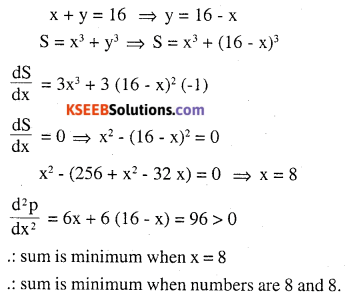Question 17.
A square piece of tin of side 18 cm ¡s to be made into a box without top, by cutting a square from each corner and folding up the flaps to form the box. What should be the side of the square to be cut off so that the volume of the box is the maximum possible.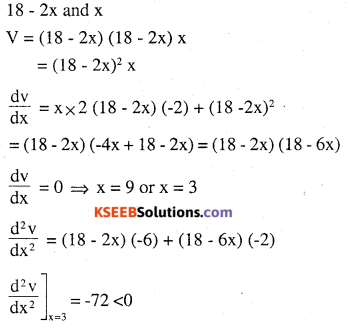x = 9 is not possible, it so side = 0
volume is maximum when the sides are 12,12,3
volume =12 x 12 x 3 = 432 cm3
The volume is maximum when the side of the square to be cut off is 3cm.

Question 18.
A rectangular sheet of tin 45 cm by 24 cm is to be made into a box without top, by cutting off square from each corner and folding up the flaps. What should be the side of the square to be cut off so that the volume of the box is maximum ?
The sides of the box are x, 45 – 24, 45 – 2x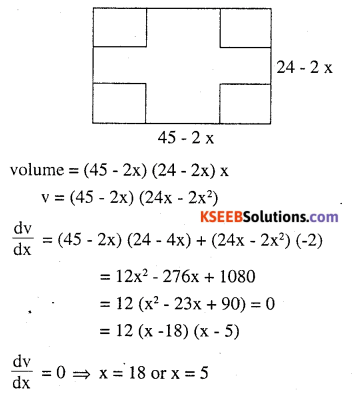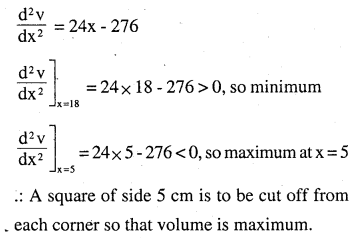Question 19.
Show that of all the rectangles inscribed in a given fixed circle, the square has the maximum area.
Let the sides of rectangle be x and y and radius of the circle is r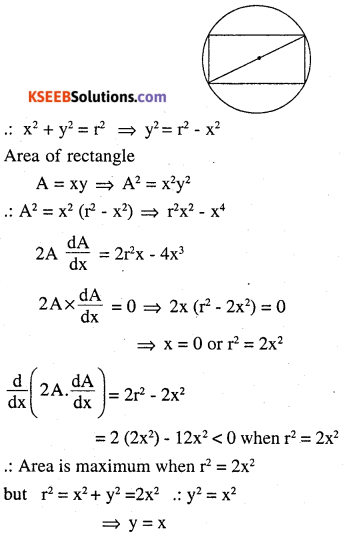hence rectangle becomes square.
∴ The area is maximum when the rectangle is a square.

Question 20.
Show that the right circular cylinder of given surface and maximum volume is such that its height is equal to the diameter of the base.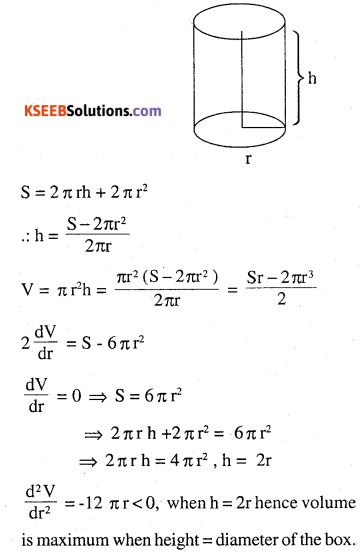Question 21.
Of all the closed cylindrical cans (right circular), of a given volume of 100 cubic centimetres, find the dimensions of the can which has the minimum surface area?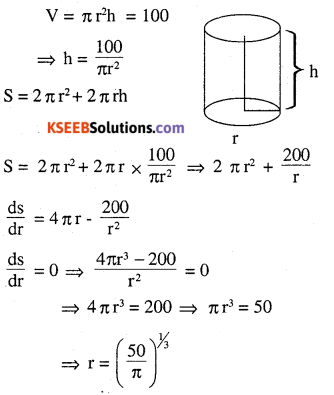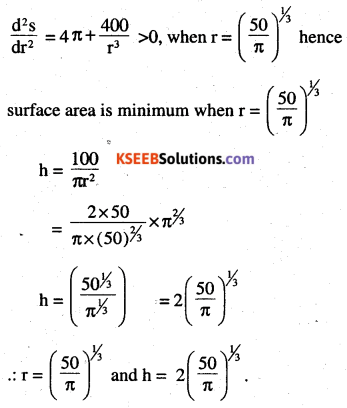Question 22.
A wire of length 28 m is to be cut into two pieces. One of the pieces is to be made into a square and the other into a circle. What should be the length of the two pieces so that the combined area of the square and the circle is minimum?Question 23.
Prove that the volume of the largest cone that can be inscribed in a sphere of radius
R is $$\frac{8}{27}$$of the volume of the sphere.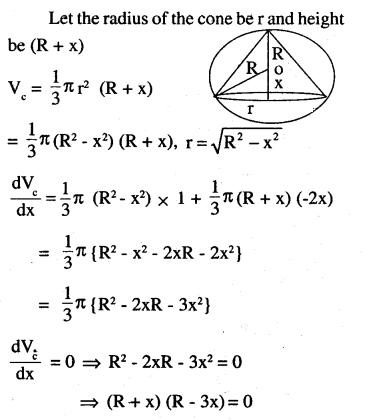Question 24.
Show that the right circular cone of least curved surface and given volume has an altitude equal to $$\sqrt{2}$$ time the radius of the base.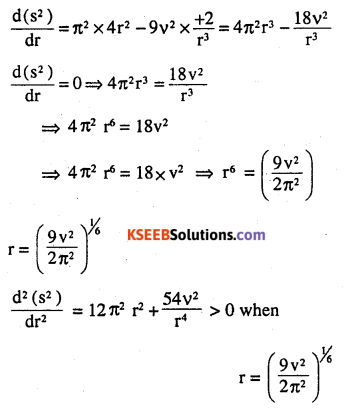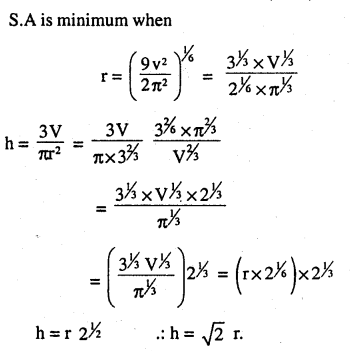Question 25.
Show that the semi-vertical angle of the cone of the maximum volume and of given slant height is $$\tan ^{-1} \sqrt{2}$$.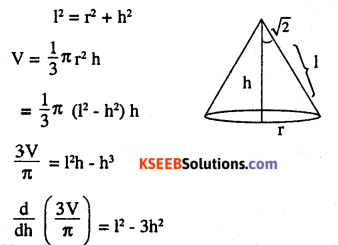Question 26.
Show that semi-vertical angle of right circular cone of given surface area and maximum volume is $$\sin ^{-1}\left(\frac{1}{3}\right)$$Choose the correct answer in the Exercises 27 and 29.

Question 27.
The point on the curve x2 = 2y which is nearest to the point (0, 5) is
(A) (2,$$\sqrt{2}$$,4)
(B) (2, $$\sqrt{2}$$, 0)
(C) (0,0)
(D) (2,2)Question 28.
For all real values of x, the minimum value of $$\frac{1-x+x^{2}}{1+x+x^{2}}$$ is
(A) 0
(B) 1
(C) 3
(D) 1/3